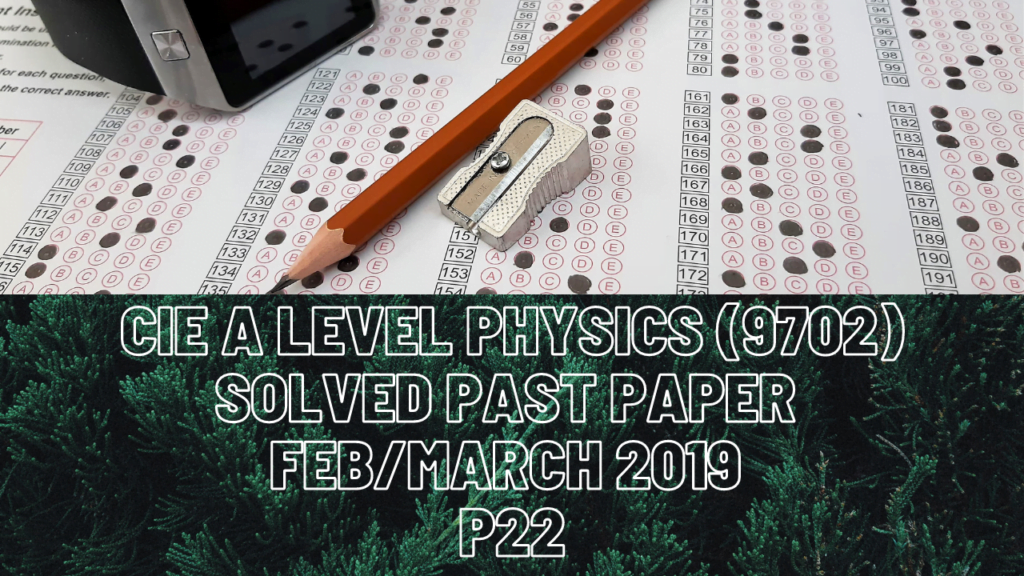A Level Physics### CIE A Level Physics Solved Past Paper Feb/March 2019 P22

2 biii) 1. Decrease in gravitational potential energy requires vertical height.

2. Force due to air resistance is going to be equal to g.p.e at A which is 2.7*10^6J because kinetic energy stays constant throughout.

3) Ball X is traveling in x-direction but ball Y is traveling at an angle which means it has a horizontal and a vertical component for momentum. Mind that if the motion is towards the left or downwards, the ball has negative momentum. Momentum before collision on either axis is equal to momentum after collision on that axis.

4 bi) 1. Since it is mentioned that direction of the field is vertically upwards, positively charged plate therefore is placed at the bottom and the negatively charged plate will be on the top.

ii) Sphere Y is negatively charged particle thus electric force acting on it is vertically downwards which makes it get attracted to the positively charged plate at the bottom.

iii) Torque is product of electric force on a sphere and perpendicular distance between both spheres. Find the perpendicular distance with the help of given torque and electric force on the sphere. Point Z is the mid point of the rod so take half of 72mm which is the rod’s length and half of the perpendicular distance to find the angle.

5 ci) Light with shorter wavelength means that this light will have decreased ratio of x/D which is also the gradient of fig.5.2. The relationship between x and D remains the same, the only thing that differs now is lower gradient so y-intercept of your new graph can be anything less than 4.0mm of x.

6 bi) 1. Current across 32ohms of resistor and parallel connection of resistor X and Y is the same so voltage across either 32 ohms divided by 32 ohms or voltage across the parallel connection divided by combined resistance of X & Y is current in the circuit.

4 & 5. Current across the parallel connection is 0.15A and when voltmeter reads 4.8V, it means p.d. across X and Y is 1.2V. This way you can combined resistance of the parallel connection followed by applying the formula of combined resistance to get the resistance of X.

ii) Resistance of X when decreases, this decreases the combined resistance of the parallel connection which in turn decrease the p.d. across X & Y. Thus voltmeter reading increases.